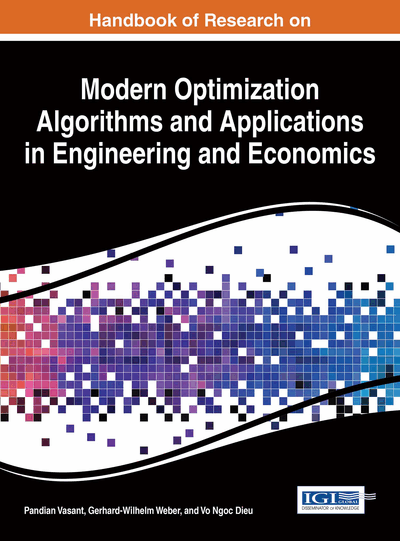# Verification of Iterative Methods for the Linear Complementarity Problem: Verification of Iterative Methods for LCPs

H. Saberi Najafi (Islamic Azad University, Lahijan Branch, Iran) and S. A. Edalatpanah (Islamic Azad University, Lahijan Branch, Iran)
DOI: 10.4018/978-1-4666-9644-0.ch021
Available
\$37.50
No Current Special Offers

## Abstract

In the present chapter, we give an overview of iterative methods for linear complementarity problems (abbreviated as LCPs). We also introduce these iterative methods for the problems based on fixed-point principle. Next, we present some new properties of preconditioned iterative methods for solving the LCPs. Convergence results of the sequence generated by these methods and also the comparison analysis between classic Gauss-Seidel method and preconditioned Gauss-Seidel (PGS) method for LCPs are established under certain conditions. Finally, the efficiency of these methods is demonstrated by numerical experiments. These results show that the mentioned models are effective in actual implementation and competitive with each other.
Chapter Preview
Top

## Introduction

(1.1) where,denotes the transpose of the vector. Note that the components of a solution (w, z) are complementary in the sense that if, then, and if, then. It may be the case thathowever.

The Linear Complementarity Problems (LCPs) is one of the fundamental problems in optimization and mathematical programming (Murty & Yu 1988; Cottle, Pang et al., 1992). The Eq.(1.1) is a general problem which unifies bounteous practical problems in various scientific computing, economics and engineering areas. For example, linear and quadratic programming (Murty & Yu, 1988), economics and Nash equilibrium point of a bi-matrix game (Gale 1960; Lemke & Howson, 1964; Lemke, 1965), invariant capital stock (Cottle, Pang et al., 1992), optimal stopping in Markov chains(Cottle, Pang et al., 1992), contact and structural mechanics (Pfeiffer & Glocker, 2000), free boundary problem for journal bearings (Lin & Cryer 1985), traffic equilibriums(Ferris & Pang, 1997), circuit simulation (Van Eijndhoven, 1986), geometry (Du Val, 1940), etc. Therefore, it deserves to pay much more attention to the researches on both theoretical properties and numerical methods about the solution of this problem. So, many direct and iterative methods have been developed for its solution; see (Murty & Yu, 1988; Cottle, Pang et al, 1992) and the references therein.

## Complete Chapter List

Search this Book:
Reset